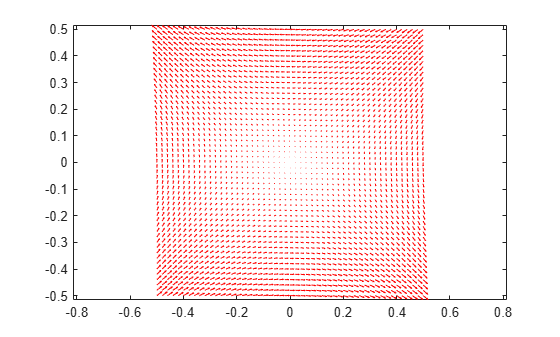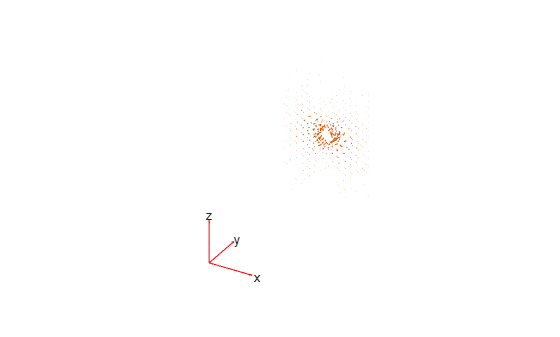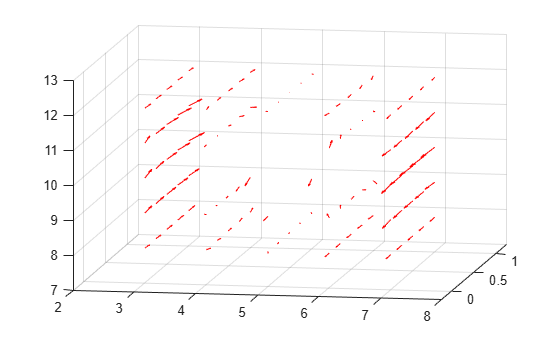# interpolateMagneticField

Interpolate magnetic field in magnetostatic result at arbitrary spatial locations

## Syntax

``Hintrp = interpolateMagneticField(magnetostaticresults,xq,yq)``
``Hintrp = interpolateMagneticField(magnetostaticresults,xq,yq,zq)``
``Hintrp = interpolateMagneticField(magnetostaticresults,querypoints)``

## Description

example

````Hintrp = interpolateMagneticField(magnetostaticresults,xq,yq)` returns the interpolated magnetic field values at the 2-D points specified in `xq` and `yq`.```

example

````Hintrp = interpolateMagneticField(magnetostaticresults,xq,yq,zq)` uses 3-D points specified in `xq`, `yq`, and `zq`.```

example

````Hintrp = interpolateMagneticField(magnetostaticresults,querypoints)` returns the interpolated magnetic field values at the points specified in `querypoints`.```

## Examples

collapse all

Create an electromagnetic model for magnetostatic analysis.

`emagmodel = createpde("electromagnetic","magnetostatic");`

Create a square geometry and include it in the model. Plot the geometry with the edge labels.

```R1 = [3,4,-1,1,1,-1,1,1,-1,-1]'; g = decsg(R1,'R1',('R1')'); geometryFromEdges(emagmodel,g); pdegplot(emagmodel,"EdgeLabels","on") xlim([-1.5 1.5]) axis equal```Specify the vacuum permeability in the SI system of units.

`emagmodel.VacuumPermeability = 1.2566370614E-6;`

Specify the relative permeability of the material.

`electromagneticProperties(emagmodel,"RelativePermeability",5000);`

Apply the magnetic potential boundary conditions on the boundaries of the square.

```electromagneticBC(emagmodel,"MagneticPotential",0,"Edge",[1 3]); electromagneticBC(emagmodel,"MagneticPotential",0.01,"Edge",[2 4]);```

Specify the current density for the entire geometry.

`electromagneticSource(emagmodel,"CurrentDensity",0.5);`

Generate the mesh.

`generateMesh(emagmodel);`

Solve the model and plot the magnetic field.

```R = solve(emagmodel); pdeplot(emagmodel,"FlowData",[R.MagneticField.Hx ... R.MagneticField.Hy]) axis equal```Interpolate the resulting magnetic field to a grid covering the central portion of the geometry, for `x` and `y` from `-0.5` to `0.5`.

```v = linspace(-0.5,0.5,51); [X,Y] = meshgrid(v); Hintrp = interpolateMagneticField(R,X,Y)```
```Hintrp = FEStruct with properties: Hx: [2601x1 double] Hy: [2601x1 double] ```

Reshape `Hintrp.Hx` and `Hintrp.Hy` and plot the resulting electric field.

```HintrpX = reshape(Hintrp.Hx,size(X)); HintrpY = reshape(Hintrp.Hy,size(Y)); figure quiver(X,Y,HintrpX,HintrpY,"Color","red")```Alternatively, you can specify the grid by using a matrix of query points.

```querypoints = [X(:),Y(:)]'; Hintrp = interpolateMagneticField(R,querypoints);```

Create an electromagnetic model for magnetostatic analysis.

`emagmodel = createpde("electromagnetic","magnetostatic");`

Import and plot the geometry representing a plate with a hole.

```importGeometry(emagmodel,"PlateHoleSolid.stl"); pdegplot(emagmodel,"FaceLabels","on","FaceAlpha",0.3)```Specify the vacuum permeability value in the SI system of units.

`emagmodel.VacuumPermeability = 1.2566370614E-6;`

Specify the relative permeability of the material.

`electromagneticProperties(emagmodel,"RelativePermeability",5000);`

Specify the current density for the entire geometry.

`electromagneticSource(emagmodel,"CurrentDensity",[0;0;0.5]);`

Apply the magnetic potential boundary conditions on the side faces and the face bordering the hole.

```electromagneticBC(emagmodel,"MagneticPotential",[0;0;0],"Face",3:6); electromagneticBC(emagmodel,"MagneticPotential",[0;0;0.01],"Face",7);```

Generate the linear mesh.

`generateMesh(emagmodel,"GeometricOrder","linear");`

Solve the model.

`R = solve(emagmodel)`
```R = MagnetostaticResults with properties: MagneticPotential: [1x1 FEStruct] MagneticField: [1x1 FEStruct] MagneticFluxDensity: [1x1 FEStruct] Mesh: [1x1 FEMesh] ```

Plot the magnetic field density.

```pdeplot3D(emagmodel,"FlowData",[R.MagneticField.Hx ... R.MagneticField.Hy ... R.MagneticField.Hz])```Interpolate the resulting magnetic field to a grid covering the central portion of the geometry, for `x`, `y`, and `z`.

```x = linspace(3,7,5); y = linspace(0,1,5); z = linspace(8,12,5); [X,Y,Z] = meshgrid(x,y,z); Hintrp = interpolateMagneticField(R,X,Y,Z)```
```Hintrp = FEStruct with properties: Hx: [125x1 double] Hy: [125x1 double] Hz: [125x1 double] ```

Reshape `Hintrp.Hx`, `Hintrp.Hy`, and `Hintrp.Hz`.

```HintrpX = reshape(Hintrp.Hx,size(X)); HintrpY = reshape(Hintrp.Hy,size(Y)); HintrpZ = reshape(Hintrp.Hz,size(Z));```

Plot the resulting magnetic field.

```figure quiver3(X,Y,Z,HintrpX,HintrpY,HintrpZ,"Color","red") view([30 10]) view([10 15])```## Input Arguments

collapse all

Solution of a magnetostatic problem, specified as a `MagnetostaticResults` object. Create `magnetostaticresults` using the `solve` function.

Example: `magnetostaticresults = solve(emagmodel)`

x-coordinate query points, specified as a real array. `interpolateMagneticField` evaluates the magnetic field at the 2-D coordinate points `[xq(i) yq(i)]` or at the 3-D coordinate points `[xq(i) yq(i) zq(i)]` for every `i`. Because of this, `xq`, `yq`, and (if present) `zq` must have the same number of entries.

`interpolateMagneticField` converts the query points to column vectors `xq(:)`, `yq(:)`, and (if present) `zq(:)`. It returns magnetic field values as a column vector of the same size. To ensure that the dimensions of the returned solution are consistent with the dimensions of the original query points, use `reshape`. For example, use `HintrpX = reshape(Hintrp.Hx,size(xq))`.

Example: `xq = [0.5 0.5 0.75 0.75]`

Data Types: `double`

y-coordinate query points, specified as a real array. `interpolateMagneticField` evaluates the magnetic field at the 2-D coordinate points `[xq(i) yq(i)]` or at the 3-D coordinate points `[xq(i) yq(i) zq(i)]` for every `i`. Because of this, `xq`, `yq`, and (if present) `zq` must have the same number of entries.

`interpolateMagneticField` converts the query points to column vectors `xq(:)`, `yq(:)`, and (if present) `zq(:)`. It returns magnetic field values as a column vector of the same size. To ensure that the dimensions of the returned solution are consistent with the dimensions of the original query points, use `reshape`. For example, use `HintrpY = reshape(Hintrp.Hy,size(yq))`.

Example: `yq = [1 2 0 0.5]`

Data Types: `double`

z-coordinate query points, specified as a real array. `interpolateMagneticField` evaluates the magnetic field at the 3-D coordinate points `[xq(i) yq(i) zq(i)]`. Therefore, `xq`, `yq`, and `zq` must have the same number of entries.

`interpolateMagneticField` converts the query points to column vectors `xq(:)`, `yq(:)`, and `zq(:)`. It returns magnetic field values as a column vector of the same size. To ensure that the dimensions of the returned solution are consistent with the dimensions of the original query points, use `reshape`. For example, use `HintrpZ = reshape(Hintrp.Hz,size(zq))`.

Example: `zq = [1 1 0 1.5]`

Data Types: `double`

Query points, specified as a real matrix with either two rows for 2-D geometry or three rows for 3-D geometry. `interpolateMagneticField` evaluates magnetic field at the coordinate points `querypoints(:,i)` for every `i`, so each column of `querypoints` contains exactly one 2-D or 3-D query point.

Example: For a 2-D geometry, ```querypoints = [0.5 0.5 0.75 0.75; 1 2 0 0.5]```

Data Types: `double`

## Output Arguments

collapse all

Magnetic field at query points, returned as an `FEStruct` object with the properties representing the spatial components of the magnetic field at the query points. For query points that are outside the geometry, `Hintrp.Hx(i)`, `Hintrp.Hy(i)`, and `Hintrp.Hz(i)` are `NaN`. Properties of an `FEStruct` object are read-only.

## Version History

Introduced in R2021a